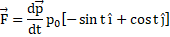# The momentum of a moving particle is vectorially gives aswherestands for time. Choose the correct option: a) The applied force is constant b) The momentum is constant c) The applied force always remains perpendicular to the momentum d) The applied force is always parallel to the momentum

## Question ID - 100519 :- The momentum of a moving particle is vectorially gives aswherestands for time. Choose the correct option: a) The applied force is constant b) The momentum is constant c) The applied force always remains perpendicular to the momentum d) The applied force is always parallel to the momentum

3537

 (c)Bothandare functions of time. So none of them is constant We see thatSoA body is rotating with angular velocity 30. If its kinetic energy is 360 J, then its moment of inertia is a) 0.8b)c)d) 1.2